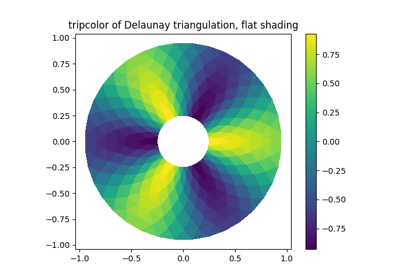# matplotlib.axes.Axes.tripcolor¶

Axes.tripcolor(ax, \*args, alpha=1.0, norm=None, cmap=None, vmin=None, vmax=None, shading='flat', facecolors=None, \*\*kwargs)

Create a pseudocolor plot of an unstructured triangular grid.

The triangulation can be specified in one of two ways; either:

tripcolor(triangulation, ...)


where triangulation is a matplotlib.tri.Triangulation object, or

tripcolor(x, y, ...)
tripcolor(x, y, triangles, ...)
tripcolor(x, y, triangles=triangles, ...)

in which case a Triangulation object will be created. See Triangulation for a explanation of these possibilities.
The next argument must be C, the array of color values, either one per point in the triangulation if color values are defined at points, or one per triangle in the triangulation if color values are defined at triangles. If there are the same number of points and triangles in the triangulation it is assumed that color values are defined at points; to force the use of color values at triangles use the kwarg facecolors=C instead of just C.
The remaining kwargs are the same as for pcolor().
## Examples using matplotlib.axes.Axes.tripcolor¶